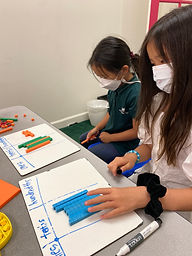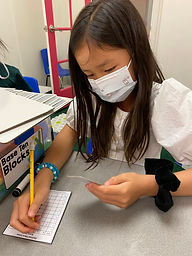## Ms. Kylie

### Target 1​

###### Lesson Type:

New

Number Operation

:

Number Reltionships

Compare numbers, recording the results of the comparison with the symbol >, =, and <.

###### 1:

Compare decimals (up to the hundredths place).

###### 2:

When comparing decimals, understand to look at the greatest place-value first.

###### 3:

Explain how a number could have a lesser value, even if it has more digits than another (i.e., 4.33<5.2).

4th

###### Vocabulary:

Equation, Hundredths, Tenths

Activities:

-Students used base ten blocks to understand the tenths and hundredths place value. They were given a decimal number and used the base ten blocks to represent the number.

-Students were given two decimal numbers to compare to see which number was more or less than.

-Students played a game of comparing decimal numbers (hundreds, tens, ones, tenths, hundredths). Each student drew one card from the deck and decided what place value to put their number in. Once they completed creating a number they compared their number to see whose number was greater.### Home Exploration

###### Guiding Questions:## Absent Students:

### Target 2

:

###### 1:

Locate points in all four quadrants of the coordinate plane.

###### 2:

Understand that the first number in a coordinate pair indicates placement on the x-axis and the second indicates placement on the y-axis.

4th

###### Vocabulary:

Coordinates, X axis, Y axis

Activities:

-Students were given a set of coordinates to graph in all four quadrants.

-Students began graphing coordinates to a mystery picture.### Home Exploration

###### Guiding Questions:### Target 3

:

###### Vocabulary:

Activities:### Home Exploration